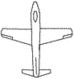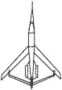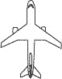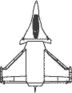# planform

(redirected from Planform area)
Also found in: Dictionary.
Related to Planform area: wing area

## planform

[′plan‚fȯrm]
(aerospace engineering)
The shape or form of an object, such as an airfoil, as seen from above, as in a plan view.
(geography)
A body of water's outline or morphology as defined by the still water line.
McGraw-Hill Dictionary of Scientific & Technical Terms, 6E, Copyright © 2003 by The McGraw-Hill Companies, Inc.

## planformStraight wingJoined wingSweptbackDelta with canard
The outline of a wing of an aircraft as viewed from above. It is the shape one would see in a blueprint or a “plan.”
References in periodicals archive ?
We divided the recorded force by the mussel's planform area to calculate tenacity.
We measured the width and height of the individuals within each group, calculated their planform areas, and summed these areas for use in calculating whole-group tenacity:
Since a separate set of simulations was not conducted for this foil, the results presented in Table 3 correspond to section lift and drag coefficients taken from the literature, wherein the chord length (Z) is used instead of the planform area (A) in (4).
Distributions of the force required to dislodge mussels (Mytilus californianus) of measured planform area were measured in March 1990 on Tatoosh Island, Washington (48 [degrees] 24' N, 124 [degrees] 44' W) by the method described by Denny (1987) and as reported in Denny (1993a).
For each species, the relationship between dislodgment force and size (measured here as planform area) was modeled using the allometric equation described previously (Eq.
Note that because of the difference in reference area, values of [S.sub.d,pl] (based on planform area) cannot be compared directly to values of [S.sub.d,pr] (based on profile area).
where C is the calculated mass of Ca[CO.sub.3] (milligrams) and A is total planform area ([cm.sup.2]) of a frond.
Here [rho] is the density of seawater (nominally 1025 kg [m.sup.-3]), U is water velocity (m [s.sup.-1]), S is maximal planform area ([m.sup.2], essentially half the wetted surface area of the frond), and [C.sub.d] is the dimensionless coefficient of drag.
The planform area of each sample was estimated using ImageJ, an image analysis program distributed by the Research Services Branch of the National Institutes of Health.
Carrington found no significant relationship between cross-sectional area of the stipe and thallus planform area (P [much greater than] 0.05), and neither did we ([r.sup.2] = 0.023, P = 0.054).
For all treatments in the canopy experiments, force exerted by one thallus (mass = 7.9 g, planform area = 0.01 [m.sup.2]) on the force beam was determined at flow speeds of 0.09, 0.18, 0.27, 0.36, and 0.45 m [s.sup.-1].
obs.), thalli similar in size ([m.sup.2]) and shape ([C.sub.D]) to those used in the present experiments occur at intertidal sites: the experimental thalli used in the present study overlap in terms of both [C.sub.D] (t-test, [P.sub.1,46] = 0.41) and planform area (t-test, [P.sub.1,46] = 0.79) with the largest thalli found in two dense intertidal canopies in Maine in the autumn (M.

Site: Follow: Share:
Open / Close